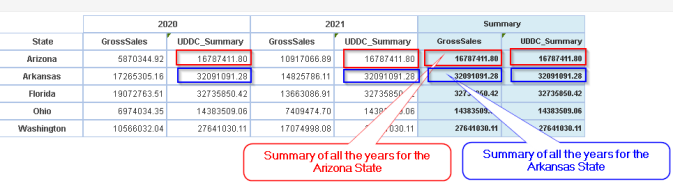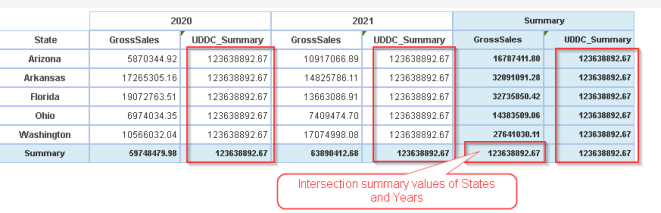• Derive summary on Row dimension

Description:

Calculate the summary value of Row dimension using summary function.

UDDC Expression:

UDDC Expression for calculating the row dimension Summary:

 summary( row Dimension, ””, Measure )

Example:

UDDC Expression for calculating the Summary of Row (State) Dimension - UDDC_Summary:

 summary(State, "", GrossSales )

As per the below example,

For the year 2020, the sum of Gross sales of all the states is 59748479.98. Hence the UDDC_Summary value is 59748479.98.

For the year 2021, the sum of Gross sales of all the states is 63890412.68. Hence the UDDC_Summary value is 63890412.68• Derive summary on Column dimension

Description:

Calculate the summary value of column dimension using summary function.

UDDC Expression:

UDDC Expression for calculating Summary:

 summary( ””, columnDimension, Measure )

Example:

UDDC Expression for calculating Summary of Column (Year) Dimension - UDDC_Summary:

 summary("", Year, GrossSales )

As per the below example,

For the State Arizona, the sum of Gross sales of all the years is 16787411.80. Hence the UDDC_Summary value is 16787411.80.

For the State Arkansas, the sum of Gross sales of all the years is 32091091.28. Hence the UDDC_Summary value is 32091091.28• Derive summary on Row and Column dimension (intersection)

Description:

Calculate the summary intersection value of row and column dimension using summary function.

 summary( rowDimension, columnDimension, Measure )

UDDC Expression:

UDDC Expression for calculating the intersection Summary value of row and column dimension (State & Year combination)- UDDC_Summary:

 summary(State, Year, GrossSales )

Example:

As per the below example,

The intersection summary value for all the states and years are 123638892.67. Hence the UDDC_Summary value is 123638892.67# Type of Summary Expression Example 1 Derive summary on Row dimension Summary( Row dimension “”, Measure ) Summary( State,“”, Grosssales ) where State is the row dimension in the crosstab on which the summary will be calculated. Since we are calculating state wise summary here, the column dimension will be empty (“”). The total gross sales of all the states will be the output. 2 Derive summary on Column dimension Summary (“”, Column Dimension,, Measure) Summary("",Year,GrossSales) where Year is the column dimension in the crosstab on which the summary will be calculated. Since we are calculating year wise summary here, the row dimension will be empty (“”). The total gross sales of all the years will be the output. 3 Derive summary on Row and Column dimension (intersection) Summary ( Row Dimension, Column Dimension, Measure ) Summary(State,Year,Grosssales) where State is the row dimension and Year is the column dimension on which the combined summary will be calculated. The total gross sales of all the states and Years are will be the output.